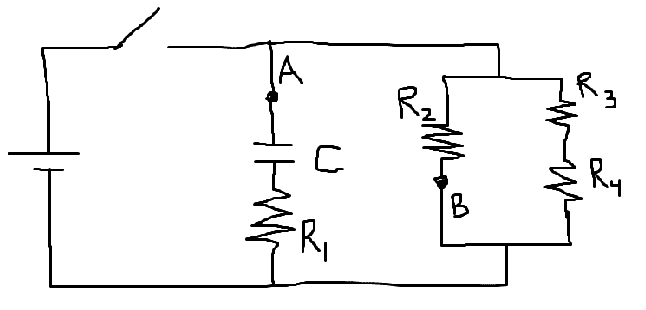# Charging and discharging RC circuits

• Archived

## Homework Statement

The RC circuit pictured below begins with capacitor C completely uncharged.

I: What is the current at point A immediately after the switch closes?
II:What is the current at point A 'at the end of the quaternary period' (I lol'd when he worded it like that) after the switch is closed?
III: 'After a few proton half-lives,' (again, lol) the switch is opened by alien explorers. Let us call this time t0. After how much additional time will the charge on capacitor C equal half its charge when the switch was first opened?
IV: At that time, what is the current at point B?

$E = 12.00V$
$C = 15.00\mu F$
$R_1 = 1000\Omega$
$R_2 = 2000\Omega$
$R_3 = 1500\Omega$
$R_4 = 1000\Omega$

(NOTE: I will be using "E" to denote the emf because I can't find a script E.)

The RC Circuit:## Homework Equations

Charging:
$q = CE(1 - e^{(\frac{-t}{RC})})$

$i = \frac{dq}{dt} = \frac{-CE}{RC} * e^{(\frac{-t}{RC})}$

Discharging:

$q = q_0 e^{(\frac{-t}{RC})}$

$i = \frac{dq}{dt} = \frac{-q_0}{RC} * e^{(\frac{-t}{RC})}$

## The Attempt at a Solution

I: Since the capacitor is uncharged at the beginning and point A is connected directly to the voltage source, the current across A is simply given by

$i_A = \frac{E}{R_1} = \frac{12V}{1000\Omega} = 0.0120A = 12.00mA$

II: Because the capacitor is fully charged by the time the next geological period commences, there is no current across point A.

III: By the relation noted above for the charge on a discharging capacitor in an open RC circuit,

$q = \frac{1}{2}q_0 = q_0 * e^{(\frac{-t}{RC})} \Rightarrow e^{(\frac{-t}{RC})} = \frac{1}{2}$

$\frac{-t}{RC} = ln(\frac{1}{2} \Rightarrow t = -RC * -ln(2) = RC * ln(2)$

$t = 1000\Omega * 1.500 * 10^{-5}F * 0.6931 = 1.040 * 10^{-2}s = 10.40ms$

IV: The current through capacitor C, resistor R1, and point A (as well as through the equivalent resistance of R2 in parallel with R3 and R4) goes as the quotient of the potential difference across the capacitor and the equivalent resistance of the circuit:

$R = R_1 + (\frac{1}{R_2} + \frac{1}{R_3 + R_4})^{-1} = 2111\Omega [itex]i = \frac{dq}{dt} = \frac{q_0}{RC} * e^{(\frac{-t}{RC})} = \frac{q_0}{RC} * e^{ln(1/2)} = \frac{q_0}{2RC} = \frac{CV_0}{2RC} = \frac{V_0}{2R} = \frac{12V}{4222\Omega} = 2.842mA = 2.842 * 10^{-3}A$

This is the part I'm less sure about:

I think that if you traverse the circuit counterclockwise (starting at C, going through R1, and then going through the parallel resistors) then the circuit follows the Kirchhoff rules. (It would follow them going clockwise as well, with the opposite signs compared to counterclockwise.)

So the potential across the capacitor is given by $V = \frac{q}{C}$. This means that the potential across the wire connecting R2 and R4 to R1 -- and thus at point B -- is given by

$V_B = q_{0}e^{ln(1/2)} - iR_1 = \frac{9.000 * 10^{-5}C}{1.500 * 10^{-5}F} - 2.842 * 10^{-3}A * 1.000 * 10^{3}\Omega = 3.158V$.

And since passing through the parallel resistors through either path leads to an equipotential surface with 0 potential, the potential drop across the R3 & R4 series and across R2 (which is in series with point B) is 3.158V. Thus,

$i_B = \frac{V_B}{R_2} = \frac{3.158V}{2000\Omega} = 1.579 * 10^{-3}A = 1.579mA$

So to summarize the results:

I: iA = 12.00mA = 1.200 * 10-2A

II: iA = 0

III: t = 10.40ms = 1.040 * 10-2s

IV: iB = 1.579mA = 1.579 * 10-3A

gneill
Mentor
For part III the capacitor will be discharging through the entire resistor network, not just R1. So the value of the time constant must take into account the entire equivalent resistance.

The equivalent resistance that the capacitor "sees" while discharging with be:

##R_{eq} = R_1 + \left[ \frac{R_2 (R_3 + R_4)}{R_2 + R_3 + R_4} \right] = 2.111~kΩ##

and the time constant becomes:

##\tau = R_{eq} C = 31.67~ms##

This gives us the time to discharge half of the charge as:

##t = ln(2) \tau = 21.95~ms##